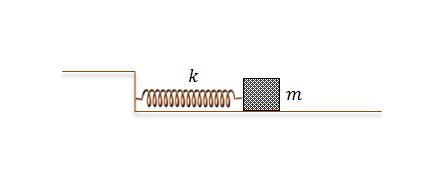Classical Mechanics

# Amplitude, Frequency, Wave Number, Phase Shift

A body of mass $$m = 2\text{ kg}$$ is oscillating on a spring. The position in meters of the body as a function of time $$t$$ can be expressed as

$x(t) = A\cos{(2\pi ft)} \text{ m} .$

If the phase of $$x(t)$$ is shifted by $$\delta = \frac{8 \pi}{5} \text{ rad},$$ the time delay of its cycle is $$KT \text{ s},$$ where $$T$$ is the period of the oscillation. What is the constant $$K?$$

A body of mass $$m = 2\text{ kg}$$ is oscillating on a spring. The position of the body in meters as a function of time $$t$$ can be expressed ass $x(t) = A\cos{(2\pi ft)} \text{ m}.$ If $$x(t)$$ is delayed by $$\frac{1}{3}$$ of its cycle, what is the phase shift $$\delta$$ in radians?As illustrated in the above diagram, a body of mass $$m = 4 \text{ kg}$$ attached to a spring with spring constant $$k = 16 \text{ N/m}$$ is oscillating on a frictionless floor. Initially at $$t=0,$$ the position of the body was $$x(t=0) = 4 \text{ m }$$ and the velocity was $$v(t=0) = 0 \text{ m/s }.$$ What is the amplitude of the oscillation?

A body of mass $$m = 2 \text{ kg}$$ is oscillating on a spring. The position of the body is given as $$x(t) = 4 \cos{(30 t)} \text{ m},$$ where $$x$$ denotes the difference in the length of the spring from its original length. What is the period of the body?

Assume that $$\pi = 3$$.

A normal human can hear the sound frequency from $$20 \text{ Hz}$$ to $$20000 \text{ Hz}.$$ Find the longest wavelength of the hearable sound, given that the speed of sound is $$340 \text{ m/s}.$$

×

Problem Loading...

Note Loading...

Set Loading...